# 机器学习|划分聚类之 K-Means 详解

## K-Means 聚类方法

 对于未聚类数据集，首先随机初始化 K 个（代表拟聚类簇个数）中心点，如图红色五角星所示。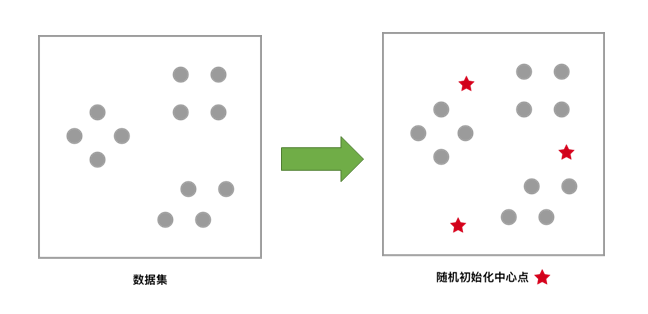每一个样本按照距离自身最近的中心点进行聚类，等效于通过两中心点连线的中垂线划分区域。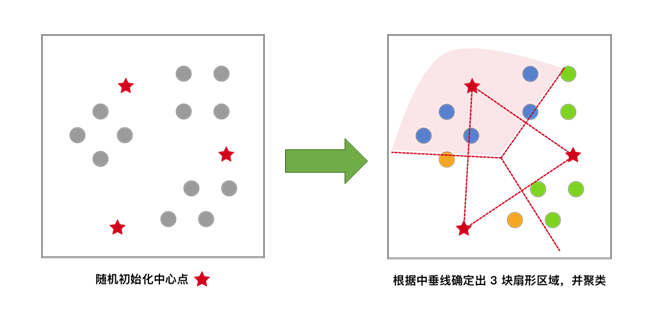依据上次聚类结果，移动中心点到个簇的质心位置，并将此质心作为新的中心点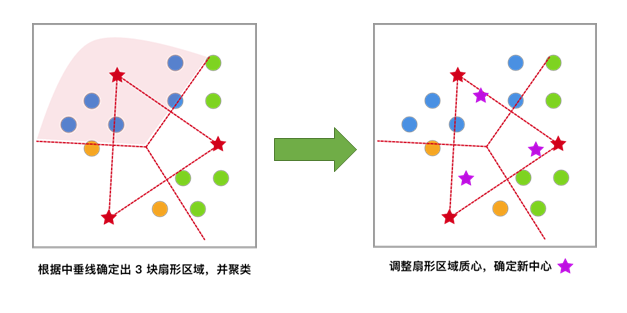反复迭代，直至中心点的变化满足收敛条件（变化很小或几乎不变化），最终得到聚类结果。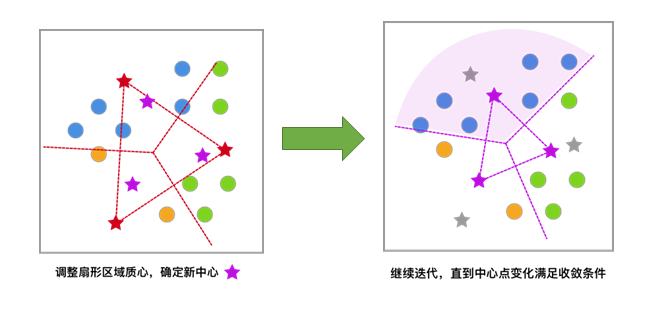### 生成示例数据

|

|

|

• n_samples：表示生成数据总个数,默认为 100 个。
• n_features：表示每一个样本的特征个数，默认为 2 个。
• centers：表示中心点的个数，默认为 3 个。
• center_box：表示每一个中心的边界,默认为 -10.0到10.0。
• random_state：表示生成数据的随机数种子。

• data：表示数据信息。
• label：表示数据类别。

|

|

|

### 数据可视化

|

|

|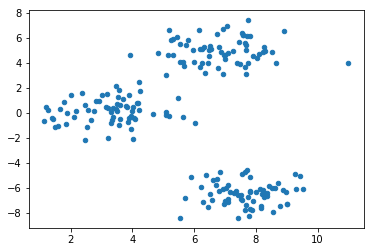### 随机初始化中心点

|

|

|

|

|

|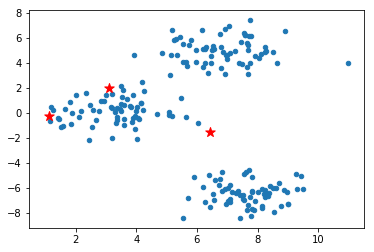### 计算样本与中心点的距离

deuc= ⎷N∑i=1(Xi−Yi)2(1)

$$(1)deuc=∑i=1N(Xi−Yi)2$$

• X$X$, Y$Y$ ：两个数据点
• N$N$：每个数据中有 N$N$ 个特征值，
• Xi$Xi$ ：数据 X$X$ 的第 i$i$ 个特征值

|

|

|

### 最小化 SSE，更新聚类中心

SSE(C)=K∑k=1∑xi∈Ck|xi−ck|2(2)

$$(2)SSE(C)=∑k=1K∑xi∈Ck|xi−ck|2$$

ck=∑xi∈CkxiI(Ck)(3)

$$(3)ck=∑xi∈CkxiI(Ck)$$

I(Ck)$I(Ck)$ 表示在第 k$k$ 个集合 Ck$Ck$ 中数据的个数。

|

|

|

### K-Means 聚类算法实现

K-Means 算法则采用的是迭代算法，避开优化 SSE 函数，通过不断移动中心点的距离，最终达到聚类的效果。

#### 算法流程

1. 初始化中心点：判断数据集可能被分为 k$k$ 个子集，随机生成 k$k$ 个随机点作为每一个子集的中心点。
2. 距离计算，类别标记：样本和每一个中心点进行距离计算，将距离最近的中心点所代表的类别标记为该样本的类别。
3. 中心点位置更新：计算每一个类别中的所有样本的均值，作为新的中心点位置。
4. 重复 2，3 步骤，直到中心点位置不再变化。

#### 算法实现

|

|

|

|

|

|

|

|

|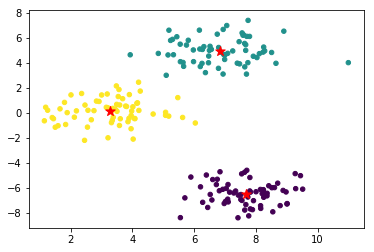### 中心点移动过程可视化

|

|

|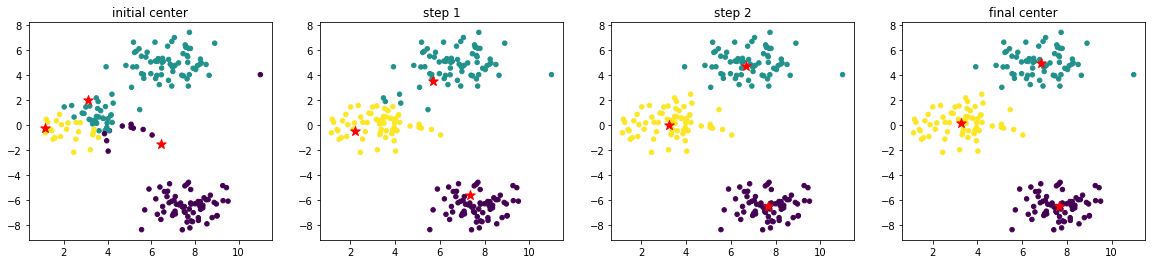Text(0.5, 1.0, ‘final center’)

• 初始化中心点的位置很好，比较均匀分布在了数据范围中。如果初始化中心点集中分布在某一角落，迭代次数肯定会增加。
• 示例数据分布规整和简单，使得无需迭代多次就能收敛。

### K-Means 算法聚类中的 K 值选择

|

|

|

• X：表示需要聚类的数据。
• n_clusters：表示聚类的个数，也就是 K 值。

• centroid：表示中心点坐标。
• label：表示聚类后每一个样本的类别。
• inertia：每一个样本与最近中心点距离的平方和，即 SSE。

|

|

|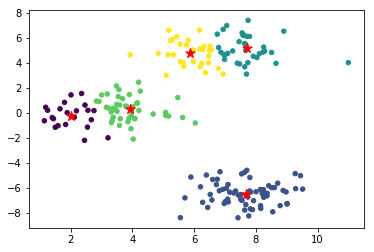|

|

|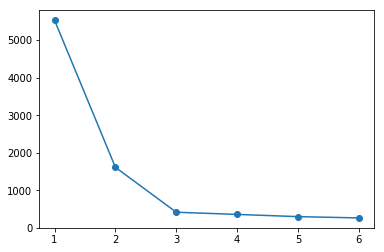## K-Means++ 聚类算法

### 生成示例数据

|

|

|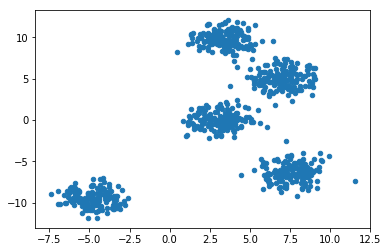### 随机初始化中心点

|

|

|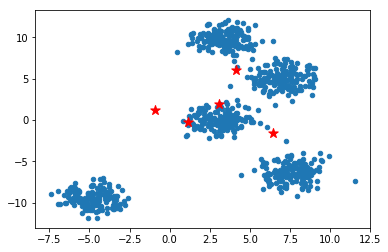### K-Means 聚类

|

|

|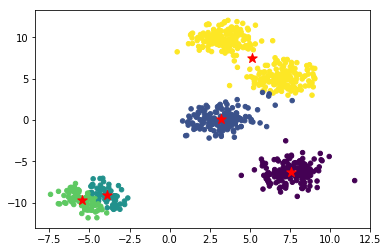|

|

|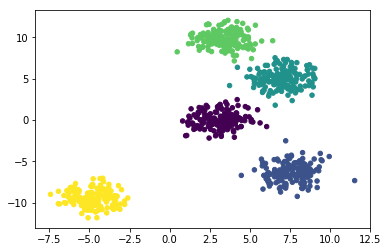### K-Means++ 算法流程

K-Means++ 相较于 K-Means 在初始化中心点上做了改进，在其他方面和 K-Means 相同。

1. 在数据集中随机选择一个样本点作为第一个初始化的聚类中心。
2. 计算样本中的非中心点与最近中心点之间的距离 D(x)$D(x)$ 并保存于一个数组里，将数组中的这些距离加起来得到 Sum(D(x))$Sum(D(x))$。
3. 取一个落在 Sum(D(x))$Sum(D(x))$范围中的随机值 R$R$ ，重复计算 R=R−D(x)$R=R−D(x)$ 直至得到 R≤0$R≤0$ ，选取此时的点作为下一个中心点。
4. 重复 2,3 步骤，直到 K$K$ 个聚类中心都被确定。
5. 对 K$K$ 个初始化的聚类中心，利用 K-Means 算法计算最终的聚类中心。

### K-Means++ 算法实现

K-Means++ 在初始化样本点之后，计算其他样本与其最近的中心点距离之和，以备下一个中心点的选择，下面用 Python 来进行实现：

|

|

|

|

|

|

|

|

|

|

|

|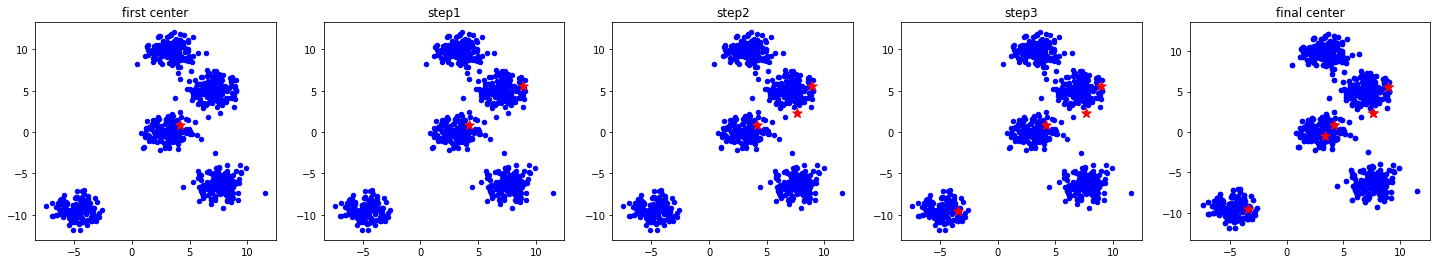Text(0.5, 1.0, ‘final center’)

|

|

|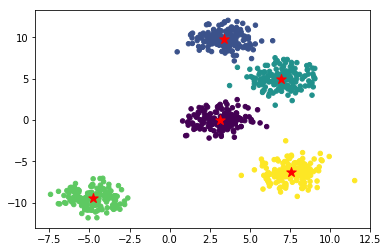## Mini-Batch K-Means 聚类算法

|

|

|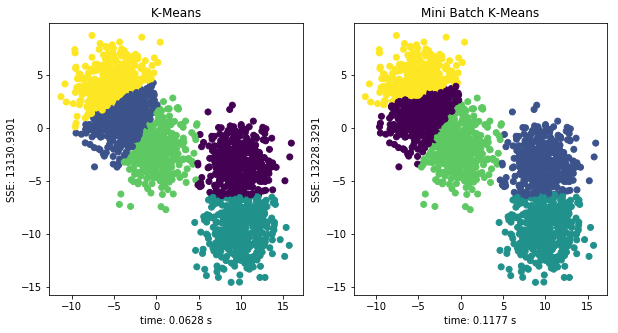Text(0.5, 1.0, ‘Mini Batch K-Means’)

http://blog.laugh12321.cn/2019/02/09/cluster_of_k-means/

laugh12321

2019-02-09

2020-10-23